# E-PolyLearning

 1. Select a figure from amongst the Answer Figures which will continue the same series as established by the five Problem Figures.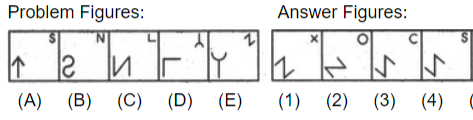a. 1 b. 2 c. 3 d. 4

 2. Select a figure from amongst the Answer Figures which will continue the same series as established by the five Problem Figures.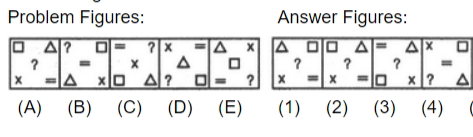a. 1 b. 2 c. 3 d. 4
 3. Select a figure from amongst the Answer Figures which will continue the same series as established by the five Problem Figures.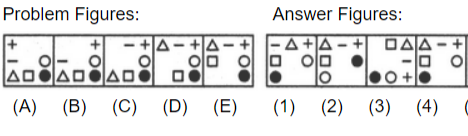a. 1 b. 2 c. 3 d. 4
 4. Select a figure from amongst the Answer Figures which will continue the same series as established by the five Problem Figures.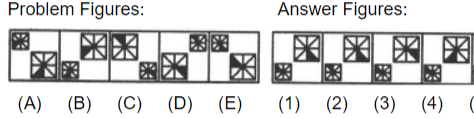a. 1 b. 2 c. 3 d. 4
 5. Select a figure from amongst the Answer Figures which will continue the same series as established by the five Problem Figures.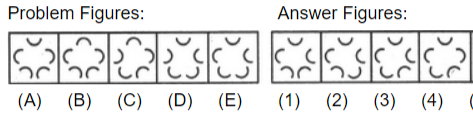a. 1 b. 2 c. 3 d. 4